احفظ التاريخ! يعود مؤتمر Google I / O من 18 إلى 20 مايو.ترجمت واجهة Cloud Translation API‏ هذه الصفحة.

# قيود الشكل باستخدام Tensorflow Lattice

## Overview

This tutorial is an overview of the constraints and regularizers provided by the TensorFlow Lattice (TFL) library. Here we use TFL canned estimators on synthetic datasets, but note that everything in this tutorial can also be done with models constructed from TFL Keras layers.

Before proceeding, make sure your runtime has all required packages installed (as imported in the code cells below).

## Setup

Installing TF Lattice package:

pip install -q tensorflow-lattice


Importing required packages:

import tensorflow as tf

from IPython.core.pylabtools import figsize
import itertools
import logging
import matplotlib
from matplotlib import pyplot as plt
import numpy as np
import pandas as pd
import sys
import tensorflow_lattice as tfl
logging.disable(sys.maxsize)


Default values used in this guide:

NUM_EPOCHS = 1000
BATCH_SIZE = 64
LEARNING_RATE=0.01


## Training Dataset for Ranking Restaurants

Imagine a simplified scenario where we want to determine whether or not users will click on a restaurant search result. The task is to predict the clickthrough rate (CTR) given input features:

• Average rating (avg_rating): a numeric feature with values in the range [1,5].
• Number of reviews (num_reviews): a numeric feature with values capped at 200, which we use as a measure of trendiness.
• Dollar rating (dollar_rating): a categorical feature with string values in the set {"D", "DD", "DDD", "DDDD"}.

Here we create a synthetic dataset where the true CTR is given by the formula:

$$CTR = 1 / (1 + exp\{\mbox{b(dollar_rating)}-\mbox{avg_rating}\times log(\mbox{num_reviews}) /4 \})$$

where $b(\cdot)$ translates each dollar_rating to a baseline value:

$$\mbox{D}\to 3,\ \mbox{DD}\to 2,\ \mbox{DDD}\to 4,\ \mbox{DDDD}\to 4.5.$$

This formula reflects typical user patterns. e.g. given everything else fixed, users prefer restaurants with higher star ratings, and "\$\$" restaurants will receive more clicks than "\$", followed by "\$\$\$" and "\$\$\$\$".

def click_through_rate(avg_ratings, num_reviews, dollar_ratings):
dollar_rating_baseline = {"D": 3, "DD": 2, "DDD": 4, "DDDD": 4.5}
return 1 / (1 + np.exp(
np.array([dollar_rating_baseline[d] for d in dollar_ratings]) -
avg_ratings * np.log1p(num_reviews) / 4))


Let's take a look at the contour plots of this CTR function.

def color_bar():
bar = matplotlib.cm.ScalarMappable(
norm=matplotlib.colors.Normalize(0, 1, True),
cmap="viridis",
)
bar.set_array([0, 1])
return bar

def plot_fns(fns, split_by_dollar=False, res=25):
"""Generates contour plots for a list of (name, fn) functions."""
num_reviews, avg_ratings = np.meshgrid(
np.linspace(0, 200, num=res),
np.linspace(1, 5, num=res),
)
if split_by_dollar:
dollar_rating_splits = ["D", "DD", "DDD", "DDDD"]
else:
dollar_rating_splits = [None]
if len(fns) == 1:
fig, axes = plt.subplots(2, 2, sharey=True, tight_layout=False)
else:
fig, axes = plt.subplots(
len(dollar_rating_splits), len(fns), sharey=True, tight_layout=False)
axes = axes.flatten()
axes_index = 0
for dollar_rating_split in dollar_rating_splits:
for title, fn in fns:
if dollar_rating_split is not None:
dollar_ratings = np.repeat(dollar_rating_split, res**2)
values = fn(avg_ratings.flatten(), num_reviews.flatten(),
dollar_ratings)
title = "{}: dollar_rating={}".format(title, dollar_rating_split)
else:
values = fn(avg_ratings.flatten(), num_reviews.flatten())
subplot = axes[axes_index]
axes_index += 1
subplot.contourf(
avg_ratings,
num_reviews,
np.reshape(values, (res, res)),
vmin=0,
vmax=1)
subplot.title.set_text(title)
subplot.set(xlabel="Average Rating")
subplot.set(ylabel="Number of Reviews")
subplot.set(xlim=(1, 5))

_ = fig.colorbar(color_bar(), cax=fig.add_axes([0.95, 0.2, 0.01, 0.6]))

figsize(11, 11)
plot_fns([("CTR", click_through_rate)], split_by_dollar=True)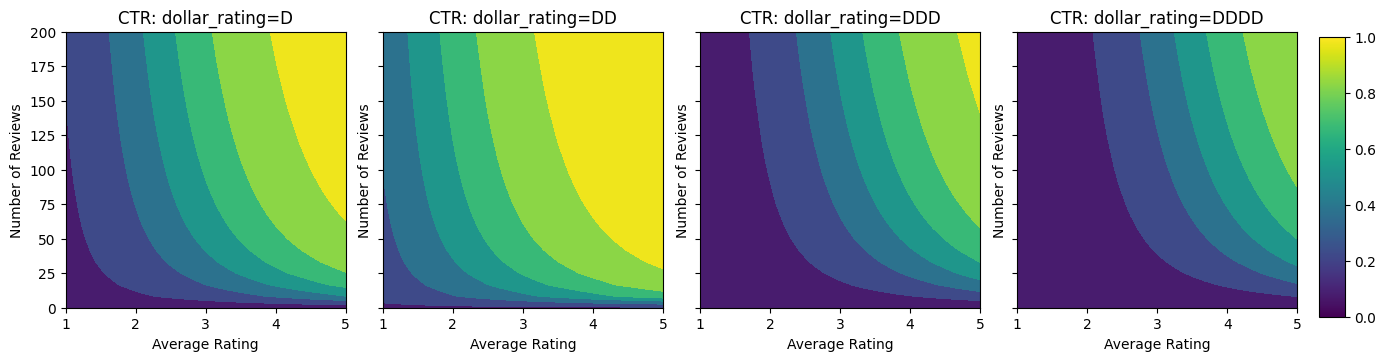### Preparing Data

We now need to create our synthetic datasets. We start by generating a simulated dataset of restaurants and their features.

def sample_restaurants(n):
avg_ratings = np.random.uniform(1.0, 5.0, n)
num_reviews = np.round(np.exp(np.random.uniform(0.0, np.log(200), n)))
dollar_ratings = np.random.choice(["D", "DD", "DDD", "DDDD"], n)
ctr_labels = click_through_rate(avg_ratings, num_reviews, dollar_ratings)
return avg_ratings, num_reviews, dollar_ratings, ctr_labels

np.random.seed(42)
avg_ratings, num_reviews, dollar_ratings, ctr_labels = sample_restaurants(2000)

figsize(5, 5)
fig, axs = plt.subplots(1, 1, sharey=False, tight_layout=False)
for rating, marker in [("D", "o"), ("DD", "^"), ("DDD", "+"), ("DDDD", "x")]:
plt.scatter(
x=avg_ratings[np.where(dollar_ratings == rating)],
y=num_reviews[np.where(dollar_ratings == rating)],
c=ctr_labels[np.where(dollar_ratings == rating)],
vmin=0,
vmax=1,
marker=marker,
label=rating)
plt.xlabel("Average Rating")
plt.ylabel("Number of Reviews")
plt.legend()
plt.xlim((1, 5))
plt.title("Distribution of restaurants")
_ = fig.colorbar(color_bar(), cax=fig.add_axes([0.95, 0.2, 0.01, 0.6]))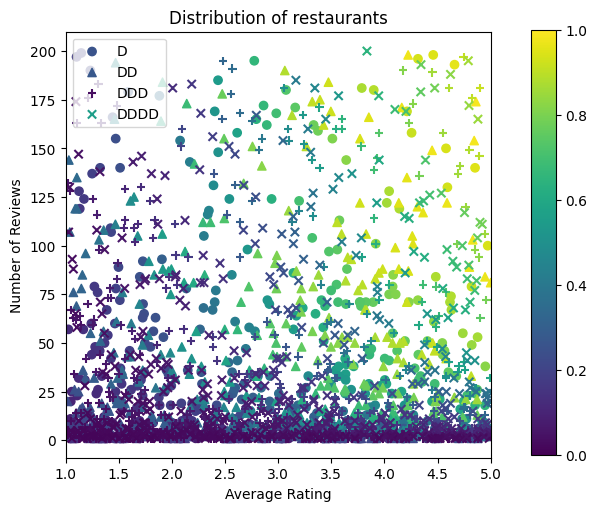Let's produce the training, validation and testing datasets. When a restaurant is viewed in the search results, we can record user's engagement (click or no click) as a sample point.

In practice, users often do not go through all search results. This means that users will likely only see restaurants already considered "good" by the current ranking model in use. As a result, "good" restaurants are more frequently impressed and over-represented in the training datasets. When using more features, the training dataset can have large gaps in "bad" parts of the feature space.

When the model is used for ranking, it is often evaluated on all relevant results with a more uniform distribution that is not well-represented by the training dataset. A flexible and complicated model might fail in this case due to overfitting the over-represented data points and thus lack generalizability. We handle this issue by applying domain knowledge to add shape constraints that guide the model to make reasonable predictions when it cannot pick them up from the training dataset.

In this example, the training dataset mostly consists of user interactions with good and popular restaurants. The testing dataset has a uniform distribution to simulate the evaluation setting discussed above. Note that such testing dataset will not be available in a real problem setting.

def sample_dataset(n, testing_set):
(avg_ratings, num_reviews, dollar_ratings, ctr_labels) = sample_restaurants(n)
if testing_set:
# Testing has a more uniform distribution over all restaurants.
num_views = np.random.poisson(lam=3, size=n)
else:
# Training/validation datasets have more views on popular restaurants.
num_views = np.random.poisson(lam=ctr_labels * num_reviews / 50.0, size=n)

return pd.DataFrame({
"avg_rating": np.repeat(avg_ratings, num_views),
"num_reviews": np.repeat(num_reviews, num_views),
"dollar_rating": np.repeat(dollar_ratings, num_views),
"clicked": np.random.binomial(n=1, p=np.repeat(ctr_labels, num_views))
})

# Generate datasets.
np.random.seed(42)
data_train = sample_dataset(500, testing_set=False)
data_val = sample_dataset(500, testing_set=False)
data_test = sample_dataset(500, testing_set=True)

# Plotting dataset densities.
figsize(12, 5)
fig, axs = plt.subplots(1, 2, sharey=False, tight_layout=False)
for ax, data, title in [(axs, data_train, "training"),
(axs, data_test, "testing")]:
_, _, _, density = ax.hist2d(
x=data["avg_rating"],
y=data["num_reviews"],
bins=(np.linspace(1, 5, num=21), np.linspace(0, 200, num=21)),
density=True,
cmap="Blues",
)
ax.set(xlim=(1, 5))
ax.set(ylim=(0, 200))
ax.set(xlabel="Average Rating")
ax.set(ylabel="Number of Reviews")
ax.title.set_text("Density of {} examples".format(title))
_ = fig.colorbar(density, ax=ax)Defining input_fns used for training and evaluation:

train_input_fn = tf.compat.v1.estimator.inputs.pandas_input_fn(
x=data_train,
y=data_train["clicked"],
batch_size=BATCH_SIZE,
num_epochs=NUM_EPOCHS,
shuffle=False,
)

# feature_analysis_input_fn is used for TF Lattice estimators.
feature_analysis_input_fn = tf.compat.v1.estimator.inputs.pandas_input_fn(
x=data_train,
y=data_train["clicked"],
batch_size=BATCH_SIZE,
num_epochs=1,
shuffle=False,
)

val_input_fn = tf.compat.v1.estimator.inputs.pandas_input_fn(
x=data_val,
y=data_val["clicked"],
batch_size=BATCH_SIZE,
num_epochs=1,
shuffle=False,
)

test_input_fn = tf.compat.v1.estimator.inputs.pandas_input_fn(
x=data_test,
y=data_test["clicked"],
batch_size=BATCH_SIZE,
num_epochs=1,
shuffle=False,
)


Let's start off with only two features: avg_rating and num_reviews.

We create a few auxillary functions for plotting and calculating validation and test metrics.

def analyze_two_d_estimator(estimator, name):
# Extract validation metrics.
metric = estimator.evaluate(input_fn=val_input_fn)
print("Validation AUC: {}".format(metric["auc"]))
metric = estimator.evaluate(input_fn=test_input_fn)
print("Testing AUC: {}".format(metric["auc"]))

def two_d_pred(avg_ratings, num_reviews):
results = estimator.predict(
tf.compat.v1.estimator.inputs.pandas_input_fn(
x=pd.DataFrame({
"avg_rating": avg_ratings,
"num_reviews": num_reviews,
}),
shuffle=False,
))
return [x["logistic"] for x in results]

def two_d_click_through_rate(avg_ratings, num_reviews):
return np.mean([
click_through_rate(avg_ratings, num_reviews,
np.repeat(d, len(avg_ratings)))
for d in ["D", "DD", "DDD", "DDDD"]
],
axis=0)

figsize(11, 5)
plot_fns([("{} Estimated CTR".format(name), two_d_pred),
("CTR", two_d_click_through_rate)],
split_by_dollar=False)


We can fit TensorFlow gradient boosted decision trees on the dataset:

feature_columns = [
tf.feature_column.numeric_column("num_reviews"),
tf.feature_column.numeric_column("avg_rating"),
]
gbt_estimator = tf.estimator.BoostedTreesClassifier(
feature_columns=feature_columns,
# Hyper-params optimized on validation set.
n_batches_per_layer=1,
max_depth=2,
n_trees=50,
learning_rate=0.05,
config=tf.estimator.RunConfig(tf_random_seed=42),
)
gbt_estimator.train(input_fn=train_input_fn)
analyze_two_d_estimator(gbt_estimator, "GBT")

Validation AUC: 0.6333084106445312
Testing AUC: 0.774649977684021Even though the model has captured the general shape of the true CTR and has decent validation metrics, it has counter-intuitive behavior in several parts of the input space: the estimated CTR decreases as the average rating or number of reviews increase. This is due to a lack of sample points in areas not well-covered by the training dataset. The model simply has no way to deduce the correct behaviour solely from the data.

To solve this issue, we enforce the shape constraint that the model must output values monotonically increasing with respect to both the average rating and the number of reviews. We will later see how to implement this in TFL.

## Fitting a DNN

We can repeat the same steps with a DNN classifier. We can observe a similar pattern: not having enough sample points with small number of reviews results in nonsensical extrapolation. Note that even though the validation metric is better than the tree solution, the testing metric is much worse.

feature_columns = [
tf.feature_column.numeric_column("num_reviews"),
tf.feature_column.numeric_column("avg_rating"),
]
dnn_estimator = tf.estimator.DNNClassifier(
feature_columns=feature_columns,
# Hyper-params optimized on validation set.
hidden_units=[16, 8, 8],
config=tf.estimator.RunConfig(tf_random_seed=42),
)
dnn_estimator.train(input_fn=train_input_fn)
analyze_two_d_estimator(dnn_estimator, "DNN")

Validation AUC: 0.6696228981018066
Testing AUC: 0.750156044960022## Shape Constraints

TensorFlow Lattice (TFL) is focused on enforcing shape constraints to safeguard model behavior beyond the training data. These shape constraints are applied to TFL Keras layers. Their details can be found in our JMLR paper.

In this tutorial we use TF canned estimators to cover various shape constraints, but note that all these steps can be done with models created from TFL Keras layers.

As with any other TensorFlow estimator, TFL canned estimators use feature columns to define the input format and use a training input_fn to pass in the data. Using TFL canned estimators also requires:

• a model config: defining the model architecture and per-feature shape constraints and regularizers.
• a feature analysis input_fn: a TF input_fn passing data for TFL initialization.

For a more thorough description, please refer to the canned estimators tutorial or the API docs.

### Monotonicity

We first address the monotonicity concerns by adding monotonicity shape constraints to both features.

To instruct TFL to enforce shape constraints, we specify the constraints in the feature configs. The following code shows how we can require the output to be monotonically increasing with respect to both num_reviews and avg_rating by setting monotonicity="increasing".

feature_columns = [
tf.feature_column.numeric_column("num_reviews"),
tf.feature_column.numeric_column("avg_rating"),
]
model_config = tfl.configs.CalibratedLatticeConfig(
feature_configs=[
tfl.configs.FeatureConfig(
name="num_reviews",
lattice_size=2,
monotonicity="increasing",
pwl_calibration_num_keypoints=20,
),
tfl.configs.FeatureConfig(
name="avg_rating",
lattice_size=2,
monotonicity="increasing",
pwl_calibration_num_keypoints=20,
)
])
tfl_estimator = tfl.estimators.CannedClassifier(
feature_columns=feature_columns,
model_config=model_config,
feature_analysis_input_fn=feature_analysis_input_fn,
config=tf.estimator.RunConfig(tf_random_seed=42),
)
tfl_estimator.train(input_fn=train_input_fn)
analyze_two_d_estimator(tfl_estimator, "TF Lattice")

Validation AUC: 0.6565533876419067
Testing AUC: 0.7784258723258972Using a CalibratedLatticeConfig creates a canned classifier that first applies a calibrator to each input (a piece-wise linear function for numeric features) followed by a lattice layer to non-linearly fuse the calibrated features. We can use tfl.visualization to visualize the model. In particular, the following plot shows the two trained calibrators included in the canned classifier.

def save_and_visualize_lattice(tfl_estimator):
saved_model_path = tfl_estimator.export_saved_model(
"/tmp/TensorFlow_Lattice_101/",
feature_spec=tf.feature_column.make_parse_example_spec(
feature_columns)))
model_graph = tfl.estimators.get_model_graph(saved_model_path)
figsize(8, 8)
tfl.visualization.draw_model_graph(model_graph)
return model_graph

_ = save_and_visualize_lattice(tfl_estimator)With the constraints added, the estimated CTR will always increase as the average rating increases or the number of reviews increases. This is done by making sure that the calibrators and the lattice are monotonic.

### Diminishing Returns

Diminishing returns means that the marginal gain of increasing a certain feature value will decrease as we increase the value. In our case we expect that the num_reviews feature follows this pattern, so we can configure its calibrator accordingly. Notice that we can decompose diminishing returns into two sufficient conditions:

• the calibrator is monotonicially increasing, and
• the calibrator is concave.
feature_columns = [
tf.feature_column.numeric_column("num_reviews"),
tf.feature_column.numeric_column("avg_rating"),
]
model_config = tfl.configs.CalibratedLatticeConfig(
feature_configs=[
tfl.configs.FeatureConfig(
name="num_reviews",
lattice_size=2,
monotonicity="increasing",
pwl_calibration_convexity="concave",
pwl_calibration_num_keypoints=20,
),
tfl.configs.FeatureConfig(
name="avg_rating",
lattice_size=2,
monotonicity="increasing",
pwl_calibration_num_keypoints=20,
)
])
tfl_estimator = tfl.estimators.CannedClassifier(
feature_columns=feature_columns,
model_config=model_config,
feature_analysis_input_fn=feature_analysis_input_fn,
config=tf.estimator.RunConfig(tf_random_seed=42),
)
tfl_estimator.train(input_fn=train_input_fn)
analyze_two_d_estimator(tfl_estimator, "TF Lattice")
_ = save_and_visualize_lattice(tfl_estimator)

Validation AUC: 0.6409633755683899
Testing AUC: 0.7891247272491455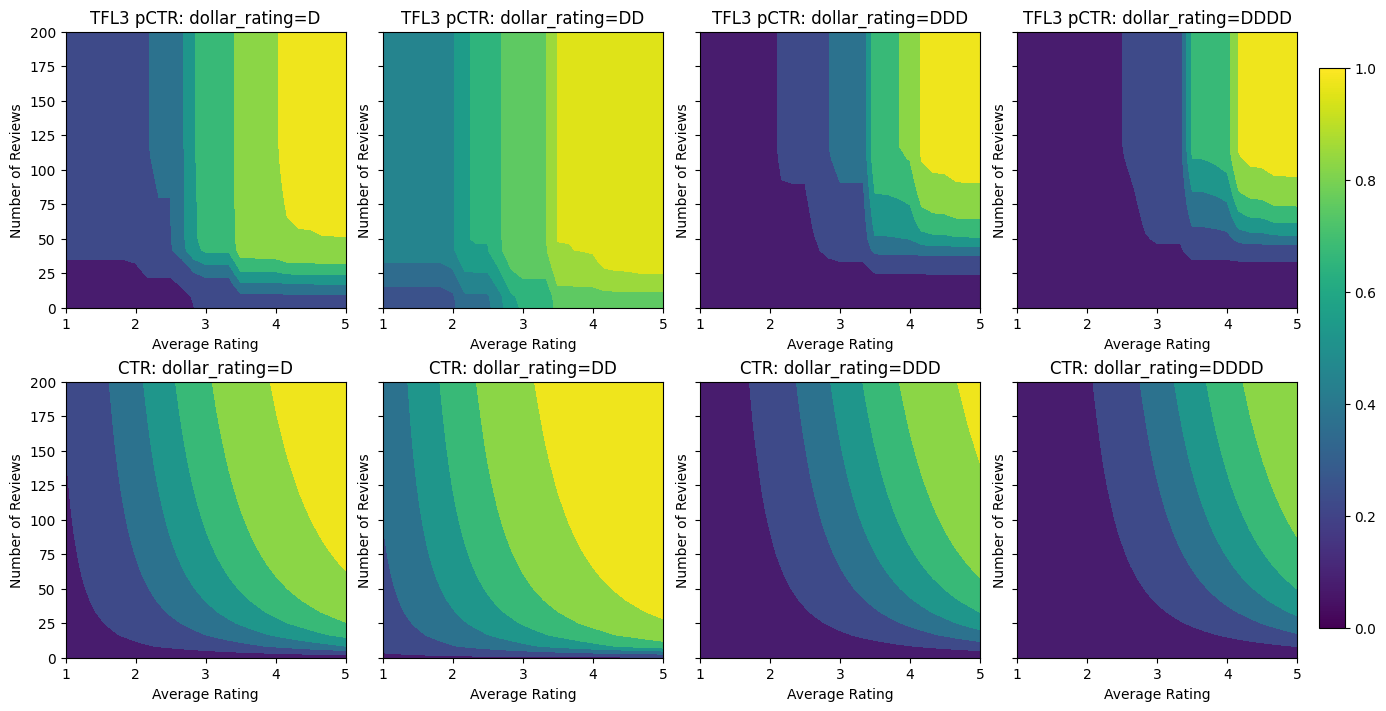Notice how the testing metric improves by adding the concavity constraint. The prediction plot also better resembles the ground truth.

### 2D Shape Constraint: Trust

A 5-star rating for a restaurant with only one or two reviews is likely an unreliable rating (the restaurant might not actually be good), whereas a 4-star rating for a restaurant with hundreds of reviews is much more reliable (the restaurant is likely good in this case). We can see that the number of reviews of a restaurant affects how much trust we place in its average rating.

We can exercise TFL trust constraints to inform the model that the larger (or smaller) value of one feature indicates more reliance or trust of another feature. This is done by setting reflects_trust_in configuration in the feature config.

feature_columns = [
tf.feature_column.numeric_column("num_reviews"),
tf.feature_column.numeric_column("avg_rating"),
]
model_config = tfl.configs.CalibratedLatticeConfig(
feature_configs=[
tfl.configs.FeatureConfig(
name="num_reviews",
lattice_size=2,
monotonicity="increasing",
pwl_calibration_convexity="concave",
pwl_calibration_num_keypoints=20,
# Larger num_reviews indicating more trust in avg_rating.
reflects_trust_in=[
tfl.configs.TrustConfig(
feature_name="avg_rating", trust_type="edgeworth"),
],
),
tfl.configs.FeatureConfig(
name="avg_rating",
lattice_size=2,
monotonicity="increasing",
pwl_calibration_num_keypoints=20,
)
])
tfl_estimator = tfl.estimators.CannedClassifier(
feature_columns=feature_columns,
model_config=model_config,
feature_analysis_input_fn=feature_analysis_input_fn,
config=tf.estimator.RunConfig(tf_random_seed=42),
)
tfl_estimator.train(input_fn=train_input_fn)
analyze_two_d_estimator(tfl_estimator, "TF Lattice")
model_graph = save_and_visualize_lattice(tfl_estimator)

Validation AUC: 0.6409633755683899
Testing AUC: 0.7891043424606323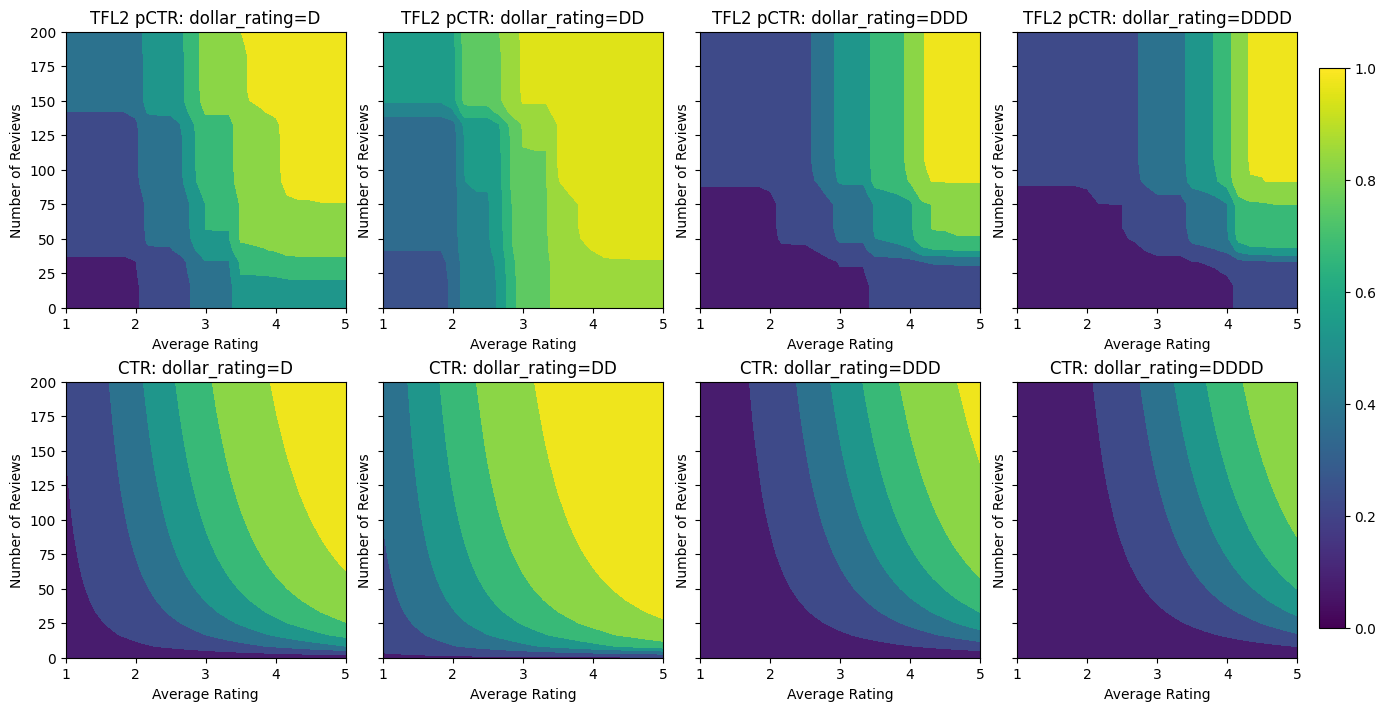The following plot presents the trained lattice function. Due to the trust constraint, we expect that larger values of calibrated num_reviews would force higher slope with respect to calibrated avg_rating, resulting in a more significant move in the lattice output.

lat_mesh_n = 12
lat_mesh_x, lat_mesh_y = tfl.test_utils.two_dim_mesh_grid(
lat_mesh_n**2, 0, 0, 1, 1)
lat_mesh_fn = tfl.test_utils.get_hypercube_interpolation_fn(
model_graph.output_node.weights.flatten())
lat_mesh_z = [
lat_mesh_fn([lat_mesh_x.flatten()[i],
lat_mesh_y.flatten()[i]]) for i in range(lat_mesh_n**2)
]
trust_plt = tfl.visualization.plot_outputs(
(lat_mesh_x, lat_mesh_y),
{"Lattice Lookup": lat_mesh_z},
figsize=(6, 6),
)
trust_plt.title("Trust")
trust_plt.xlabel("Calibrated avg_rating")
trust_plt.ylabel("Calibrated num_reviews")
trust_plt.show()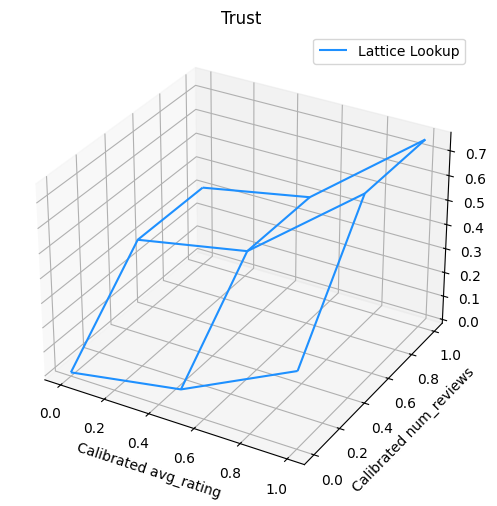### Smoothing Calibrators

Let's now take a look at the calibrator of avg_rating. Though it is monotonically increasing, the changes in its slopes are abrupt and hard to interpret. That suggests we might want to consider smoothing this calibrator using a regularizer setup in the regularizer_configs.

Here we apply a wrinkle regularizer to reduce changes in the curvature. You can also use the laplacian regularizer to flatten the calibrator and the hessian regularizer to make it more linear.

feature_columns = [
tf.feature_column.numeric_column("num_reviews"),
tf.feature_column.numeric_column("avg_rating"),
]
model_config = tfl.configs.CalibratedLatticeConfig(
feature_configs=[
tfl.configs.FeatureConfig(
name="num_reviews",
lattice_size=2,
monotonicity="increasing",
pwl_calibration_convexity="concave",
pwl_calibration_num_keypoints=20,
regularizer_configs=[
tfl.configs.RegularizerConfig(name="calib_wrinkle", l2=1.0),
],
reflects_trust_in=[
tfl.configs.TrustConfig(
feature_name="avg_rating", trust_type="edgeworth"),
],
),
tfl.configs.FeatureConfig(
name="avg_rating",
lattice_size=2,
monotonicity="increasing",
pwl_calibration_num_keypoints=20,
regularizer_configs=[
tfl.configs.RegularizerConfig(name="calib_wrinkle", l2=1.0),
],
)
])
tfl_estimator = tfl.estimators.CannedClassifier(
feature_columns=feature_columns,
model_config=model_config,
feature_analysis_input_fn=feature_analysis_input_fn,
config=tf.estimator.RunConfig(tf_random_seed=42),
)
tfl_estimator.train(input_fn=train_input_fn)
analyze_two_d_estimator(tfl_estimator, "TF Lattice")
_ = save_and_visualize_lattice(tfl_estimator)

Validation AUC: 0.6465646028518677
Testing AUC: 0.7948372960090637The calibrators are now smooth, and the overall estimated CTR better matches the ground truth. This is reflected both in the testing metric and in the contour plots.

### Partial Monotonicity for Categorical Calibration

So far we have been using only two of the numeric features in the model. Here we will add a third feature using a categorical calibration layer. Again we start by setting up helper functions for plotting and metric calculation.

def analyze_three_d_estimator(estimator, name):
# Extract validation metrics.
metric = estimator.evaluate(input_fn=val_input_fn)
print("Validation AUC: {}".format(metric["auc"]))
metric = estimator.evaluate(input_fn=test_input_fn)
print("Testing AUC: {}".format(metric["auc"]))

def three_d_pred(avg_ratings, num_reviews, dollar_rating):
results = estimator.predict(
tf.compat.v1.estimator.inputs.pandas_input_fn(
x=pd.DataFrame({
"avg_rating": avg_ratings,
"num_reviews": num_reviews,
"dollar_rating": dollar_rating,
}),
shuffle=False,
))
return [x["logistic"] for x in results]

figsize(11, 22)
plot_fns([("{} Estimated CTR".format(name), three_d_pred),
("CTR", click_through_rate)],
split_by_dollar=True)


To involve the third feature, dollar_rating, we should recall that categorical features require a slightly different treatment in TFL, both as a feature column and as a feature config. Here we enforce the partial monotonicity constraint that outputs for "DD" restaurants should be larger than "D" restaurants when all other inputs are fixed. This is done using the monotonicity setting in the feature config.

feature_columns = [
tf.feature_column.numeric_column("num_reviews"),
tf.feature_column.numeric_column("avg_rating"),
tf.feature_column.categorical_column_with_vocabulary_list(
"dollar_rating",
vocabulary_list=["D", "DD", "DDD", "DDDD"],
dtype=tf.string,
default_value=0),
]
model_config = tfl.configs.CalibratedLatticeConfig(
feature_configs=[
tfl.configs.FeatureConfig(
name="num_reviews",
lattice_size=2,
monotonicity="increasing",
pwl_calibration_convexity="concave",
pwl_calibration_num_keypoints=20,
regularizer_configs=[
tfl.configs.RegularizerConfig(name="calib_wrinkle", l2=1.0),
],
reflects_trust_in=[
tfl.configs.TrustConfig(
feature_name="avg_rating", trust_type="edgeworth"),
],
),
tfl.configs.FeatureConfig(
name="avg_rating",
lattice_size=2,
monotonicity="increasing",
pwl_calibration_num_keypoints=20,
regularizer_configs=[
tfl.configs.RegularizerConfig(name="calib_wrinkle", l2=1.0),
],
),
tfl.configs.FeatureConfig(
name="dollar_rating",
lattice_size=2,
pwl_calibration_num_keypoints=4,
# Here we only specify one monotonicity:
# D resturants has smaller value than DD restaurants
monotonicity=[("D", "DD")],
),
])
tfl_estimator = tfl.estimators.CannedClassifier(
feature_columns=feature_columns,
model_config=model_config,
feature_analysis_input_fn=feature_analysis_input_fn,
config=tf.estimator.RunConfig(tf_random_seed=42),
)
tfl_estimator.train(input_fn=train_input_fn)
analyze_three_d_estimator(tfl_estimator, "TF Lattice")
_ = save_and_visualize_lattice(tfl_estimator)

Validation AUC: 0.7699775695800781
Testing AUC: 0.8594040274620056This categorical calibrator shows the preference of the model output: DD > D > DDD > DDDD, which is consistent with our setup. Notice there is also a column for missing values. Though there is no missing feature in our training and testing data, the model provides us with an imputation for the missing value should it happen during downstream model serving.

Here we also plot the predicted CTR of this model conditioned on dollar_rating. Notice that all the constraints we required are fulfilled in each of the slices.

### Output Calibration

For all the TFL models we have trained so far, the lattice layer (indicated as "Lattice" in the model graph) directly outputs the model prediction. Sometimes we are not sure whether the lattice output should be rescaled to emit model outputs:

• the features are $log$ counts while the labels are counts.
• the lattice is configured to have very few vertices but the label distribution is relatively complicated.

In those cases we can add another calibrator between the lattice output and the model output to increase model flexibility. Here let's add a calibrator layer with 5 keypoints to the model we just built. We also add a regularizer for the output calibrator to keep the function smooth.

feature_columns = [
tf.feature_column.numeric_column("num_reviews"),
tf.feature_column.numeric_column("avg_rating"),
tf.feature_column.categorical_column_with_vocabulary_list(
"dollar_rating",
vocabulary_list=["D", "DD", "DDD", "DDDD"],
dtype=tf.string,
default_value=0),
]
model_config = tfl.configs.CalibratedLatticeConfig(
output_calibration=True,
output_calibration_num_keypoints=5,
regularizer_configs=[
tfl.configs.RegularizerConfig(name="output_calib_wrinkle", l2=0.1),
],
feature_configs=[
tfl.configs.FeatureConfig(
name="num_reviews",
lattice_size=2,
monotonicity="increasing",
pwl_calibration_convexity="concave",
pwl_calibration_num_keypoints=20,
regularizer_configs=[
tfl.configs.RegularizerConfig(name="calib_wrinkle", l2=1.0),
],
reflects_trust_in=[
tfl.configs.TrustConfig(
feature_name="avg_rating", trust_type="edgeworth"),
],
),
tfl.configs.FeatureConfig(
name="avg_rating",
lattice_size=2,
monotonicity="increasing",
pwl_calibration_num_keypoints=20,
regularizer_configs=[
tfl.configs.RegularizerConfig(name="calib_wrinkle", l2=1.0),
],
),
tfl.configs.FeatureConfig(
name="dollar_rating",
lattice_size=2,
pwl_calibration_num_keypoints=4,
# Here we only specify one monotonicity:
# D resturants has smaller value than DD restaurants
monotonicity=[("D", "DD")],
),
])
tfl_estimator = tfl.estimators.CannedClassifier(
feature_columns=feature_columns,
model_config=model_config,
feature_analysis_input_fn=feature_analysis_input_fn,
config=tf.estimator.RunConfig(tf_random_seed=42),
)
tfl_estimator.train(input_fn=train_input_fn)
analyze_three_d_estimator(tfl_estimator, "TF Lattice")
_ = save_and_visualize_lattice(tfl_estimator)

Validation AUC: 0.7697908878326416
Testing AUC: 0.861327052116394The final testing metric and plots show how using common-sense constraints can help the model avoid unexpected behaviour and extrapolate better to the entire input space.

[{ "type": "thumb-down", "id": "missingTheInformationINeed", "label":"Missing the information I need" },{ "type": "thumb-down", "id": "tooComplicatedTooManySteps", "label":"Too complicated / too many steps" },{ "type": "thumb-down", "id": "outOfDate", "label":"Out of date" },{ "type": "thumb-down", "id": "translationIssue", "label":"Translation issue" },{ "type": "thumb-down", "id": "samplesCodeIssue", "label":"Samples / code issue" },{ "type": "thumb-down", "id": "otherDown", "label":"Other" }]
[{ "type": "thumb-up", "id": "easyToUnderstand", "label":"Easy to understand" },{ "type": "thumb-up", "id": "solvedMyProblem", "label":"Solved my problem" },{ "type": "thumb-up", "id": "otherUp", "label":"Other" }]